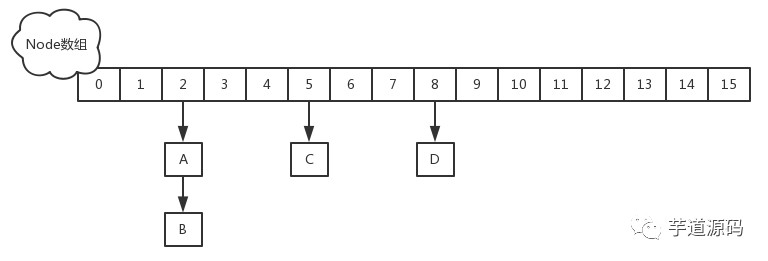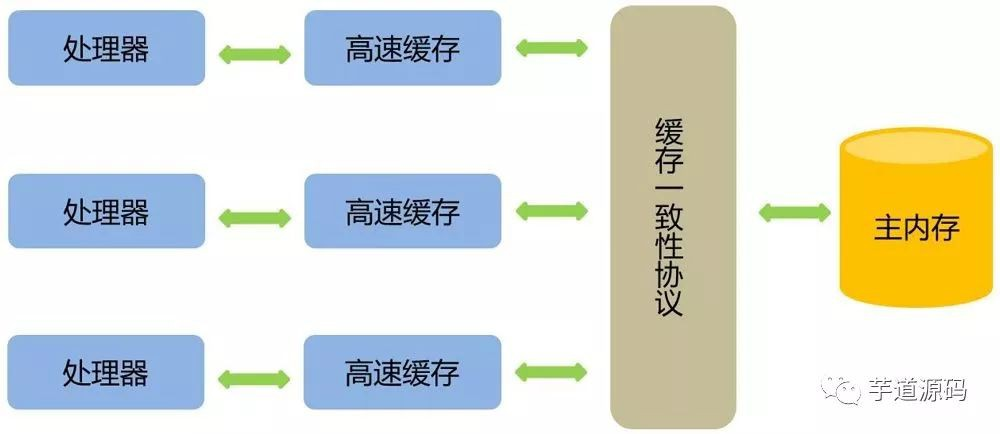# Java 經典面試題：為什麼 ConcurrentHashMap 的讀操作不需要加鎖？

• ConcurrentHashMap的簡介
• get操作原始碼
• volatile登場
• 是加在陣列上的volatile嗎?
• 用volatile修飾的Node
• 總結

# ConcurrentHashMap的簡介

• JDK1.8的實現降低鎖的粒度，JDK1.7版本鎖的粒度是基於Segment的，包含多個HashEntry，而JDK1.8鎖的粒度就是HashEntry（首節點）
• JDK1.8版本的資料結構變得更加簡單，使得操作也更加清晰流暢，因為已經使用synchronized來進行同步，所以不需要分段鎖的概念，也就不需要Segment這種資料結構了，由於粒度的降低，實現的複雜度也增加了
• JDK1.8使用紅黑樹來優化鏈表，基於長度很長的鏈表的遍歷是一個很漫長的過程，而紅黑樹的遍歷效率是很快的，代替一定閾值的鏈表，這樣形成一個最佳拍檔img

# get操作原始碼

• 首先計算hash值，定位到該table索引位置，如果是首節點符合就傳回
• 如果遇到擴容的時候，會呼叫標誌正在擴容節點ForwardingNode的find方法，查找該節點，匹配就傳回
• 以上都不符合的話，就往下遍歷節點，匹配就傳回，否則最後就傳回null
``````//會發現原始碼中沒有一處加了鎖
public V get(Object key) {
Node[] tab; Node e, p; int n, eh; K ek;
int h = spread(key.hashCode()); //計算hash
if ((tab = table) != null && (n = tab.length) > 0 &&
(e = tabAt(tab, (n - 1) & h)) != null) {//讀取首節點的Node元素
if ((eh = e.hash) == h) { //如果該節點就是首節點就傳回
if ((ek = e.key) == key || (ek != null && key.equals(ek)))
return e.val;
}
//hash值為負值表示正在擴容，這個時候查的是ForwardingNode的find方法來定位到nextTable來
//eh=-1，說明該節點是一個ForwardingNode，正在遷移，此時呼叫ForwardingNode的find方法去nextTable里找。
//eh=-2，說明該節點是一個TreeBin，此時呼叫TreeBin的find方法遍歷紅黑樹，由於紅黑樹有可能正在旋轉變色，所以find里會有讀寫鎖。
//eh>=0，說明該節點下掛的是一個鏈表，直接遍歷該鏈表即可。
else if (eh < 0)
return (p = e.find(h, key)) != null ? p.val : null;
while ((e = e.next) != null) {//既不是首節點也不是ForwardingNode，那就往下遍歷
if (e.hash == h &&
((ek = e.key) == key || (ek != null && key.equals(ek))))
return e.val;
}
}
return null;
}
``````

get沒有加鎖的話，ConcurrentHashMap是如何保證讀到的資料不是臟資料的呢？

# volatile登場

• volatile關鍵字對於基本型別的修改可以在隨後對多個執行緒的讀保持一致，但是對於取用型別如陣列，物體bean，僅僅保證取用的可見性，但並不保證取用內容的可見性。。
• 禁止進行指令重排序。

• 如果對宣告了volatile的變數進行寫操作，JVM就會向處理器發送一條指令，將這個變數所在快取行的資料寫回到系統記憶體。但是，就算寫回到記憶體，如果其他處理器快取的值還是舊的，再執行計算操作就會有問題。
• 在多處理器下，為了保證各個處理器的快取是一致的，就會實現快取一致性協議，當某個CPU在寫資料時，如果發現操作的變數是共享變數，則會通知其他CPU告知該變數的快取行是無效的，因此其他CPU在讀取該變數時，發現其無效會重新從主存中加載資料。img

# 是加在陣列上的volatile嗎?

``````/**
* The array of bins. Lazily initialized upon first insertion.
* Size is always a power of two. Accessed directly by iterators.
*/
transient volatile Node[] table;
``````

# 用volatile修飾的Node

get操作可以無鎖是由於Node的元素val和指標next是用volatile修飾的，在多執行緒環境下執行緒A修改結點的val或者新增節點的時候是對執行緒B可見的。

``````static class Node<K,V> implements Map.Entry<K,V> {
final int hash;
final K key;
//可以看到這些都用了volatile修飾
volatile V val;
volatile Node next;

Node(int hash, K key, V val, Node next) {
this.hash = hash;
this.key = key;
this.val = val;
this.next = next;
}

public final K getKey()       { return key; }
public final V getValue()     { return val; }
public final int hashCode()   { return key.hashCode() ^ val.hashCode(); }
public final String toString(){ return key + "=" + val; }
public final V setValue(V value) {
throw new UnsupportedOperationException();
}

public final boolean equals(Object o) {
Object k, v, u; Map.Entry,?> e;
return ((o instanceof Map.Entry) &&
(k = (e = (Map.Entry,?>)o).getKey()) != null &&
(v = e.getValue()) != null &&
(k == key || k.equals(key)) &&
(v == (u = val) || v.equals(u)));
}

/**
* Virtualized support for map.get(); overridden in subclasses.
*/
Node find(int h, Object k) {
Node e = this;
if (k != null) {
do {
K ek;
if (e.hash == h &&
((ek = e.key) == k || (ek != null && k.equals(ek))))
return e;
} while ((e = e.next) != null);
}
return null;
}
}
``````

# 總結

• 在1.8中ConcurrentHashMap的get操作全程不需要加鎖，這也是它比其他併發集合比如hashtable、用Collections.synchronizedMap()包裝的hashmap;安全效率高的原因之一。
• get操作全程不需要加鎖是因為Node的成員val是用volatile修飾的和陣列用volatile修飾沒有關係。
• 陣列用volatile修飾主要是保證在陣列擴容的時候保證可見性。